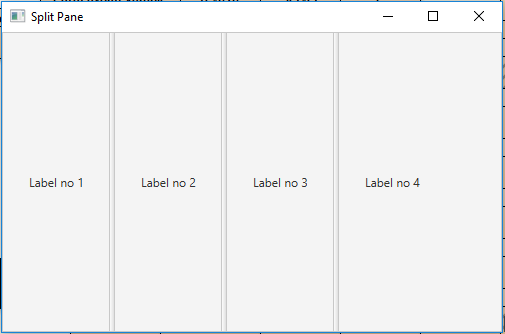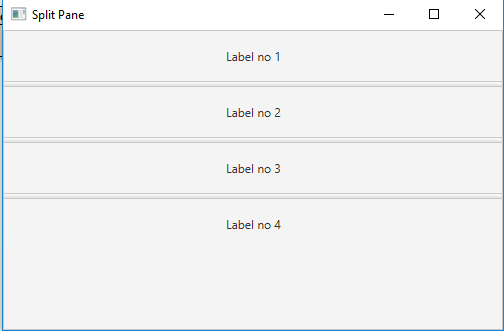# JavaFX | SplitPane Class

SplitPane class is a part of JavaFX. SplitPane class is a control which contains two or more sides separated by a divider. Sides can be dragged by the user to give more space to one of the sides which cause the other side to shrink by an equal amount. SplitPane class inherits Control class.

Constructor of the Class:

• SplitPane(): Creates a new SplitPane.
• SplitPane(Node… n): Creates a SplitPane with specified nodes.

Commonly Used Methods:

Method Explanation
getItems() Returns the items of the split pane.
getOrientation() Returns the orientation of split pane.
setDividerPosition(int dividerIndex, double position) Sets the position of divider at specified index.
setDividerPositions(double… p) Sets the position of dividers.
setOrientation(Orientation o) Sets the orienattion of splitpane.

Below programs illustrate the use of SplitPane Class:

1. Java program to create a split pane and add labels to it:
• In this program, we will create a SplitPane name split_pane.
• Create and add labels to the split pane using getItems().add() function.
• Add the split_pane to the scene and add the scene to the stage.
• Call the show() function to display the final results.

 `// Java program to create a split pane ` `// and add labels to it ` `import` `javafx.application.Application; ` `import` `javafx.scene.Scene; ` `import` `javafx.scene.control.*; ` `import` `javafx.scene.layout.*; ` `import` `javafx.stage.Stage; ` `import` `javafx.scene.layout.*; ` `import` `javafx.scene.paint.*; ` `import` `javafx.scene.text.*; ` `import` `javafx.geometry.*; ` `import` `javafx.scene.layout.*; ` `import` `javafx.scene.shape.*; ` `import` `javafx.scene.paint.*; ` `import` `javafx.scene.*; ` `import` `java.io.*; ` `import` `javafx.scene.image.*; ` ` `  `public` `class` `SplitPane_1 ``extends` `Application { ` ` `  `    ``// launch the application ` `    ``public` `void` `start(Stage stage) ` `    ``{ ` ` `  `        ``try` `{ ` ` `  `            ``// set title for the stage ` `            ``stage.setTitle(``"Split Pane"``); ` ` `  `            ``// create a splitpane ` `            ``SplitPane split_pane = ``new` `SplitPane(); ` ` `  `            ``// create labels and add it to splitPane ` `            ``for` `(``int` `i = ``1``; i < ``5``; i++) { ` ` `  `                ``split_pane.getItems().add(``new` `Label(``"\tLabel no "`  `                                                    ``+ i + ``"\t"``)); ` `            ``} ` ` `  `            ``// create a scene ` `            ``Scene scene = ``new` `Scene(split_pane, ``500``, ``300``); ` ` `  `            ``// set the scene ` `            ``stage.setScene(scene); ` ` `  `            ``stage.show(); ` `        ``} ` ` `  `        ``catch` `(Exception e) { ` ` `  `            ``System.out.println(e.getMessage()); ` `        ``} ` `    ``} ` ` `  `    ``// Main Method ` `    ``public` `static` `void` `main(String args[]) ` `    ``{ ` ` `  `        ``// launch the application ` `        ``launch(args); ` `    ``} ` `} `

Output:2. Java program to create a split pane set its orientation and add labels to it:
• In this program we will create a SplitPane name split_pane.
• Create and add labels to the split pane using getItems().add() function.
• Add the split_pane to the scene and add the scene to the stage.
• Set the orientation of the split_pane using the setOrientation() function.
• Call the show() function to display the final results.

 `// Java program to create a split pane, set ` `// its orientation and add labels to it ` `import` `javafx.application.Application; ` `import` `javafx.scene.Scene; ` `import` `javafx.scene.control.*; ` `import` `javafx.scene.layout.*; ` `import` `javafx.stage.Stage; ` `import` `javafx.scene.layout.*; ` `import` `javafx.scene.paint.*; ` `import` `javafx.scene.text.*; ` `import` `javafx.geometry.*; ` `import` `javafx.scene.layout.*; ` `import` `javafx.scene.shape.*; ` `import` `javafx.scene.paint.*; ` `import` `javafx.scene.*; ` `import` `java.io.*; ` `import` `javafx.scene.image.*; ` ` `  `public` `class` `SplitPane_2 ``extends` `Application { ` ` `  `    ``// launch the application ` `    ``public` `void` `start(Stage stage) ` `    ``{ ` ` `  `        ``try` `{ ` ` `  `            ``// set title for the stage ` `            ``stage.setTitle(``"Split Pane"``); ` ` `  `            ``// create a splitpane ` `            ``SplitPane split_pane = ``new` `SplitPane(); ` ` `  `            ``// create labels and add it to splitPane ` `            ``for` `(``int` `i = ``1``; i < ``5``; i++) { ` ` `  `                ``// create a label ` `                ``Label label = ``new` `Label(``"\tLabel no "` `+ i + ``"\t"``); ` ` `  `                ``// set preferred height ` `                ``label.setPrefHeight(``50``); ` ` `  `                ``split_pane.getItems().add(label); ` `            ``} ` ` `  `            ``// set Orientation of splitpane ` `            ``split_pane.setOrientation(Orientation.VERTICAL); ` ` `  `            ``// create a scene ` `            ``Scene scene = ``new` `Scene(split_pane, ``500``, ``300``); ` ` `  `            ``// set the scene ` `            ``stage.setScene(scene); ` ` `  `            ``stage.show(); ` `        ``} ` ` `  `        ``catch` `(Exception e) { ` ` `  `            ``System.out.println(e.getMessage()); ` `        ``} ` `    ``} ` ` `  `    ``// Main Method ` `    ``public` `static` `void` `main(String args[]) ` `    ``{ ` ` `  `        ``// launch the application ` `        ``launch(args); ` `    ``} ` `} `

Output:Note: The above programs might not run in an online IDE please use an offline compiler.

Attention reader! Don’t stop learning now. Get hold of all the important DSA concepts with the DSA Self Paced Course at a student-friendly price and become industry ready.

My Personal Notes arrow_drop_upCheck out this Author's contributed articles.

If you like GeeksforGeeks and would like to contribute, you can also write an article using contribute.geeksforgeeks.org or mail your article to contribute@geeksforgeeks.org. See your article appearing on the GeeksforGeeks main page and help other Geeks.

Please Improve this article if you find anything incorrect by clicking on the "Improve Article" button below.

Article Tags :
Practice Tags :

Be the First to upvote.

Please write to us at contribute@geeksforgeeks.org to report any issue with the above content.